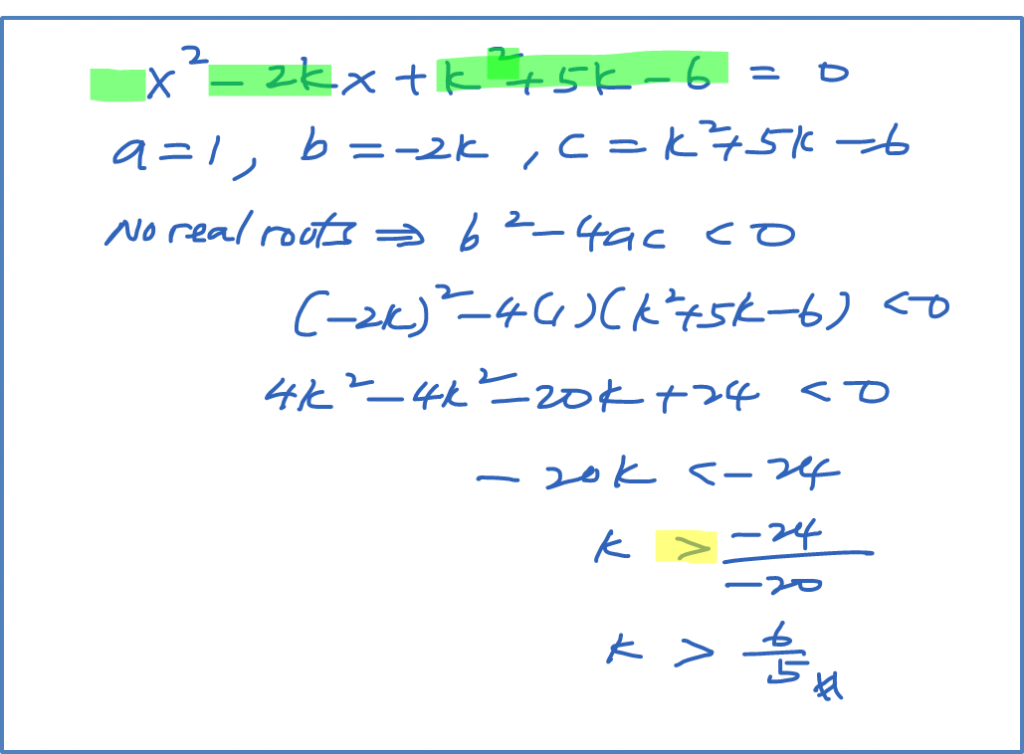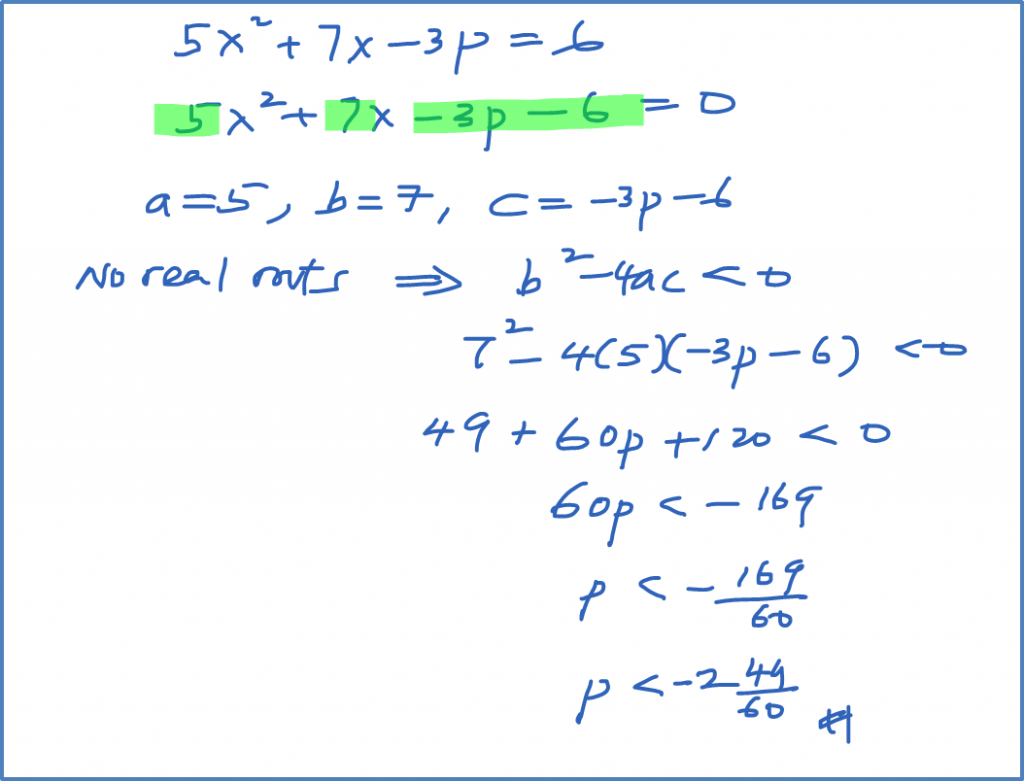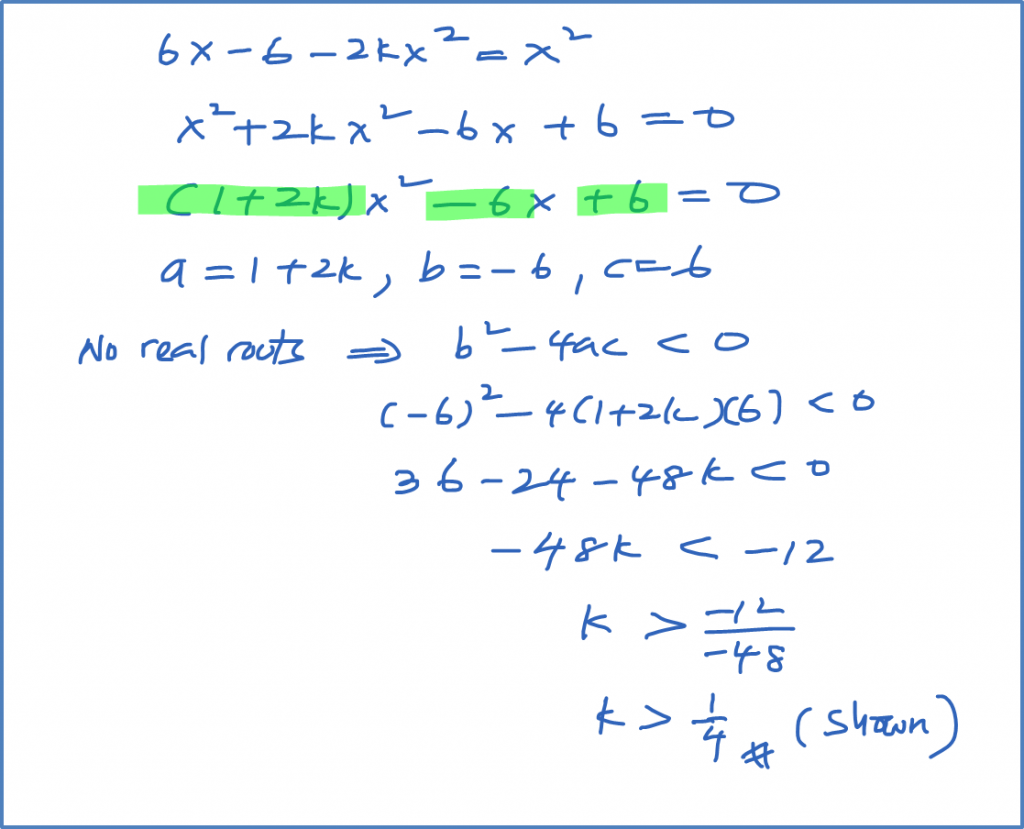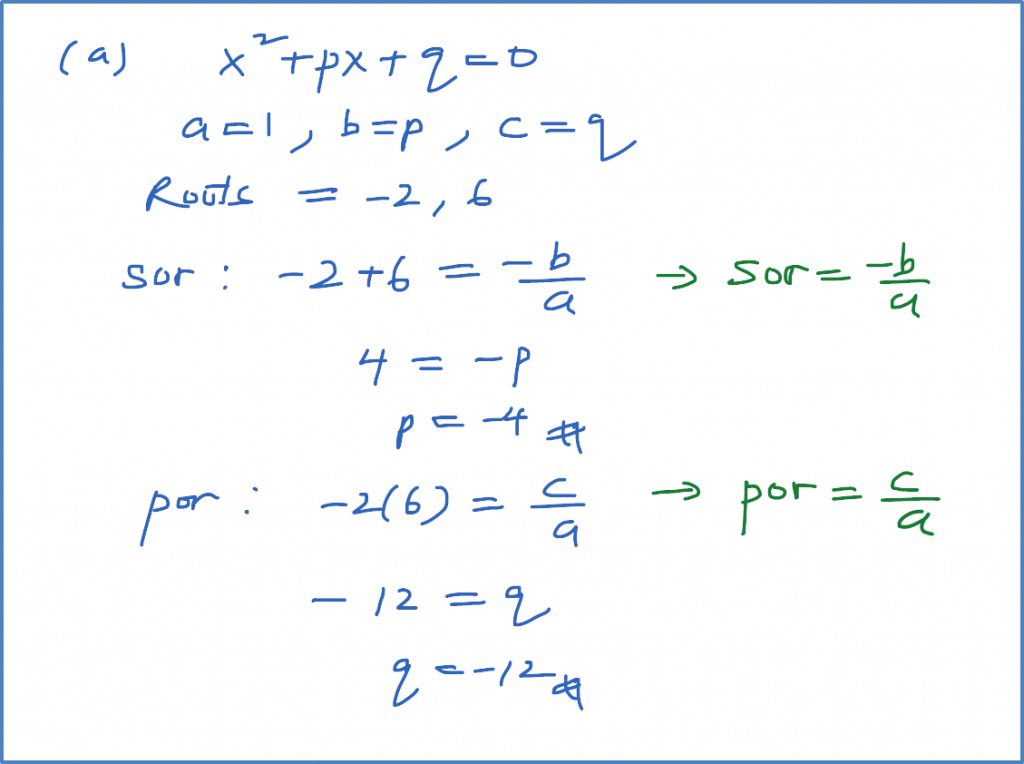# 2.9.3 Quadratic Equation, SPM Practice (Paper 1)

Question 11:
The quadratic equation ${x}^{2}-4x-1=2p\left(x-5\right)$ , where p is a constant, has two equal roots. Calculate the possible values of p.

Solution:Question 12:
Find the range of values of k for which the equation ${x}^{2}-2kx+{k}^{2}+5k-6=0$ has no real roots.

Solution:Question 13:
Find the range of values of p for which the equation $5{x}^{2}+7x-3p=6$ has no real roots.

Solution:Question 14:

Solution:Question 15:
The quadratic equation ${x}^{2}+px+q=0$ has roots –2 and 6. Find
(a) the value of p and of q,
(b) the range of values of r for which the equation ${x}^{2}+px+q=r$ has no real roots.

Solution: